# 7.4. Lesson: Spatial Statistics¶

Note

Lesson developed by Linfiniti and S Motala (Cape Peninsula University of Technology)

Spatial statistics allow you to analyze and understand what is going on in a given vector dataset. QGIS includes several standard tools for statistical analysis which prove useful in this regard.

The goal for this lesson: To know how to use QGIS’ spatial statistics tools within the Processing toolbox.

## 7.4.1.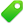Follow Along: Create a Test Dataset¶

In order to get a point dataset to work with, we’ll create a random set of points.

To do so, you’ll need a polygon dataset defining the extents of the area you want to create the points in.

We’ll use the area covered by streets.

1. Start a new project.

2. Add your roads layer, as well as the srtm_41_19 raster file (elevation data) found in `exercise_data/raster/SRTM/`.

Note

You might find that your SRTM DEM layer has a different CRS to that of the roads layer. QGIS is reprojecting both layers in a single CRS. For the following exercises this difference does not matter, but feel free to reproject a layer in another CRS as shown in this module.

3. Open Processing toolbox.

4. Use the Vector Geometry ‣ Minimum bounding geometry tool to generate an area enclosing all the roads by selecting `Convex Hull` as the Geometry Type parameter: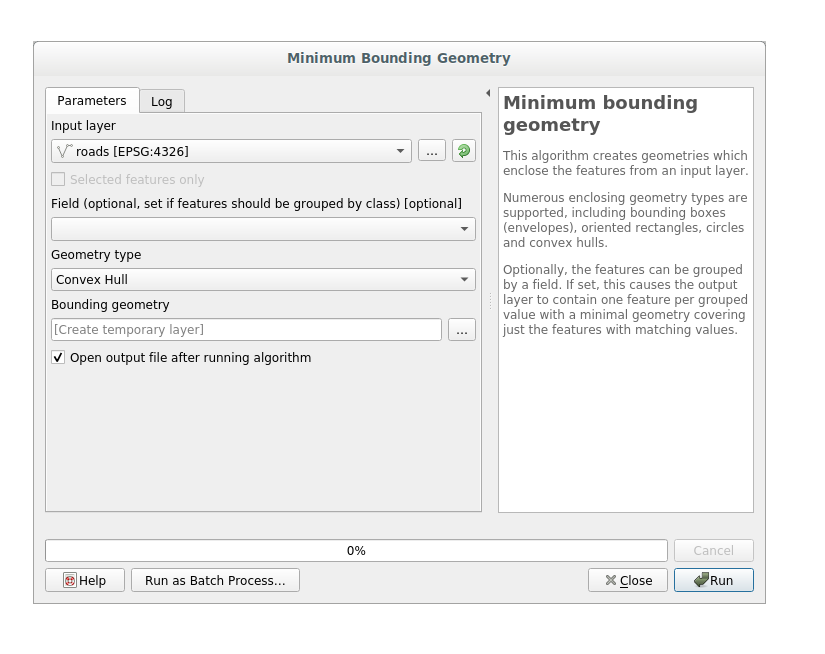As you know, if you don’t specify the output, Processing creates temporary layers. It is up to you to save the layers immediately or in a second moment.

### 7.4.1.1. Creating random points¶

• Create random points in this area using the tool at Vector Creation ‣ Random points in layer bounds: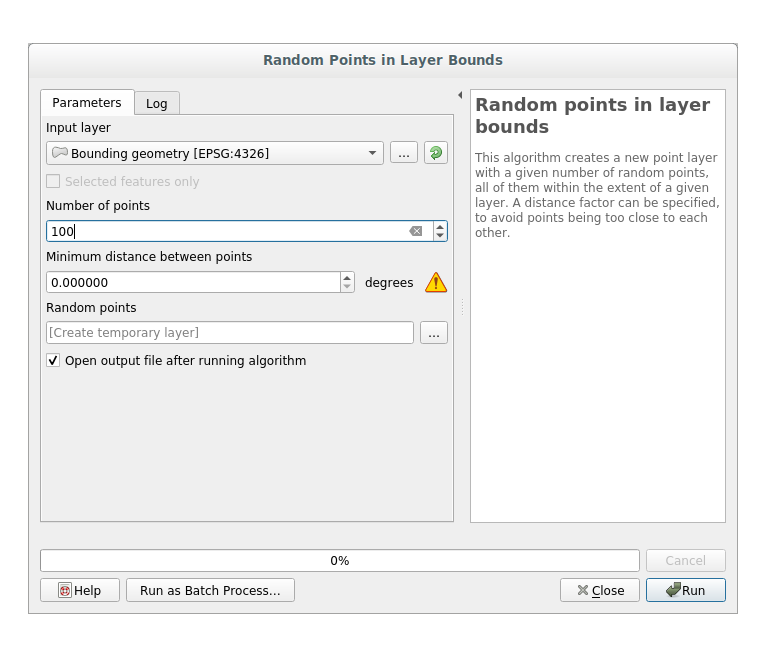Note

The yellow warning sign is telling you that that parameter concerns something about the distance. The Bounding geometry layer is in a Geographical Coordinate System and the algorithm is just reminding you this. For this example we won’t use this parameter so you can ignore it.

If needed, move the generated random point at the top of the legend to see them better: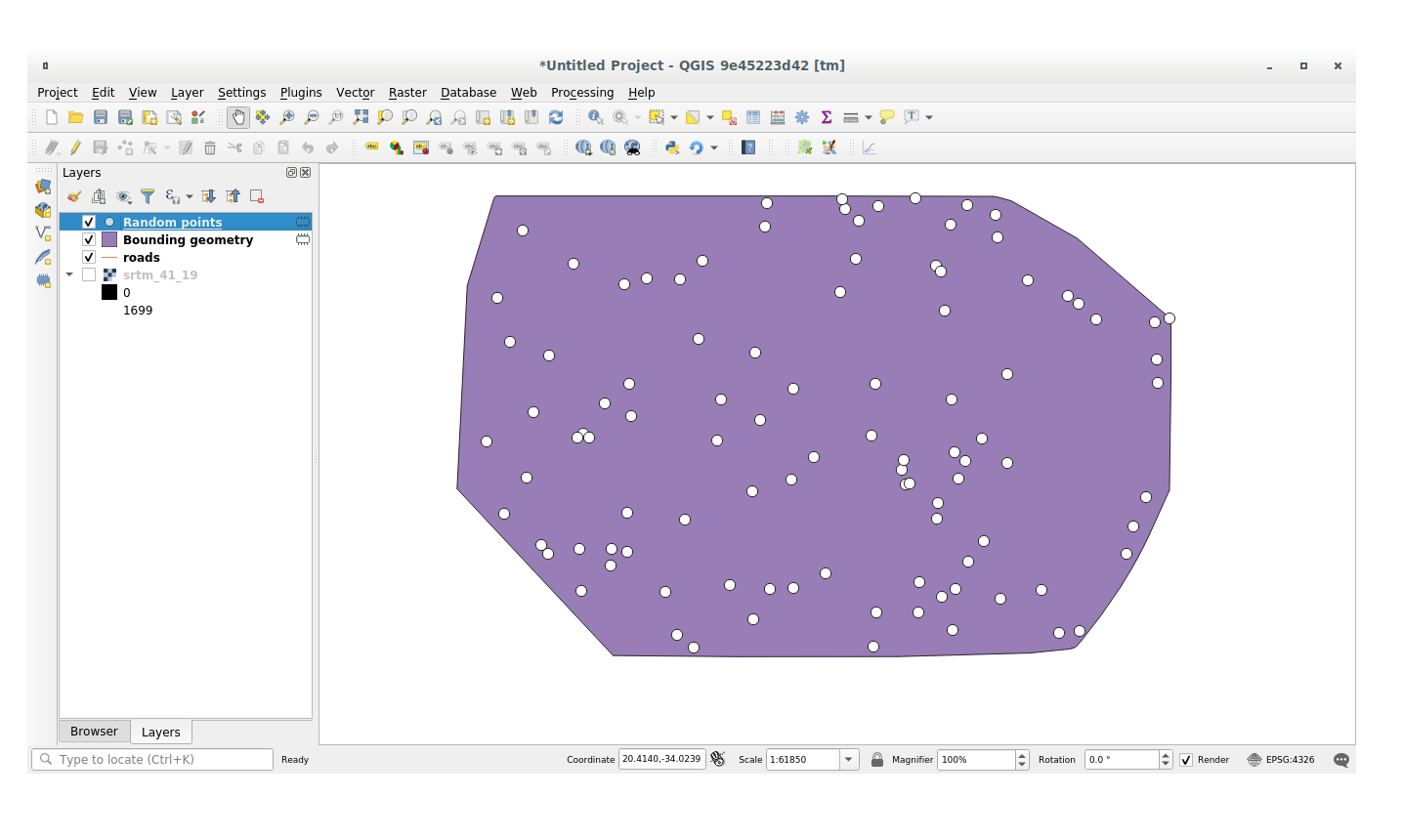### 7.4.1.2. Sampling the data¶

To create a sample dataset from the raster, you’ll need to use the Raster Analysis ‣ Sample raster values algorithm within Processing toolbox. This tool samples the raster at the points locations and copies the raster values in other field(s) depending on how many bands the raster is made of.

1. Open the Sample raster values algorithm dialog

2. Select random_points as the layer containing sampling points, and the SRTM raster as the band to get values from. The default name of the new field is `rvalue_N`, where `N` is the number of the raster band. You can change the name of the prefix if you want: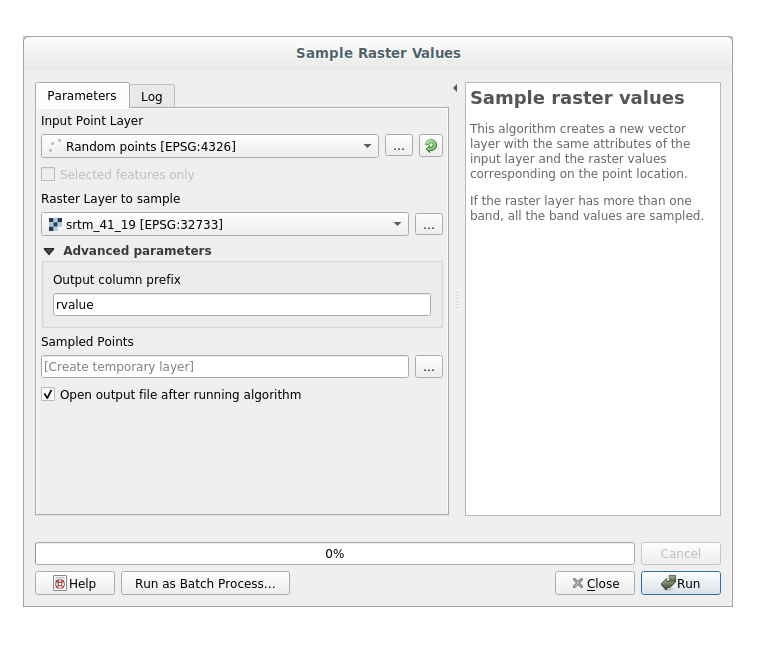3. Press Run

Now you can check the sampled data from the raster file in the attributes table of the Random points layer, they will be in a new field with the name you have chosen.

A possible sample layer is shown here: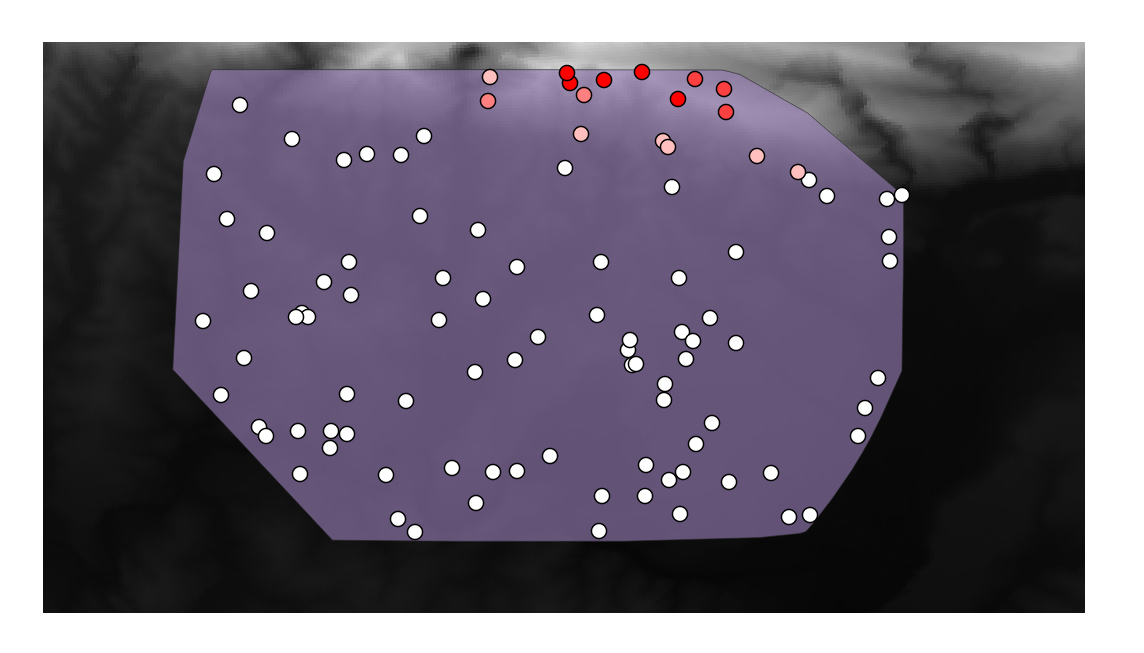The sample points are classified by their `rvalue_1` field such that red points are at a higher altitude.

You’ll be using this sample layer for the rest of the statistical exercises.

## 7.4.2.Follow Along: Basic Statistics¶

Now get the basic statistics for this layer.

1. Click on the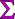icon in the Attributes Toolbar of QGIS main dialog. A new panel will pop up.

2. In the dialog that appears, specify the Sampled Points layer as the source.

3. Select the rvalue_1 field in the field combo box which is the field you will calculate statistics for.

4. The Statistics Panel will be automatically updated with the calculated statistics: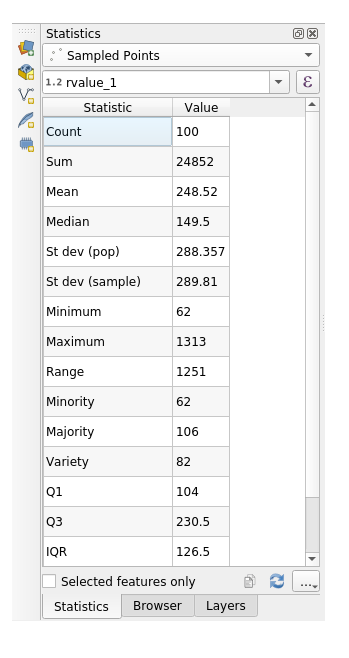Note

You can copy the values by clicking on the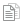Copy Statistics To Clipboard button and paste the results into a spreadsheet.

5. Close the Statistics Panel when done.

Many different statistics are available, below some description:

Count

The amount of samples/values.

Sum

Mean

The mean (average) value is simply the sum of the values divided by the amount of values.

Median

If you arrange all the values from least to greatest, the middle value (or the average of the two middle values, if N is an even number) is the median of the values.

St Dev (pop)

The standard deviation. Gives an indication of how closely the values are clustered around the mean. The smaller the standard deviation, the closer values tend to be to the mean.

Minimum

The minimum value.

Maximum

The maximum value.

Range

The difference between the minimum and maximum values.

Q1

First quartile of the data.

Q3

Third quartile of the data.

Missing (null) values

Total count of values with missing data-

## 7.4.3.Follow Along: Compute statistics on distances between points using the Distance Matrix tool¶

1. Create a new point layer as a `Temporary layer`.

2. Enter edit mode and digitize three points somewhere among the other points.

Alternatively, use the same random point generation method as before, but specify only three points.

3. Save your new layer as distance_points in the format you prefer.

To generate statistics on the distances between points in the two layers:

1. Open the tool Vector Analysis ‣ Distance matrix.

2. Select the distance_points layer as the input layer, and the Sampled Points layer as the target layer.

3. Set it up like this: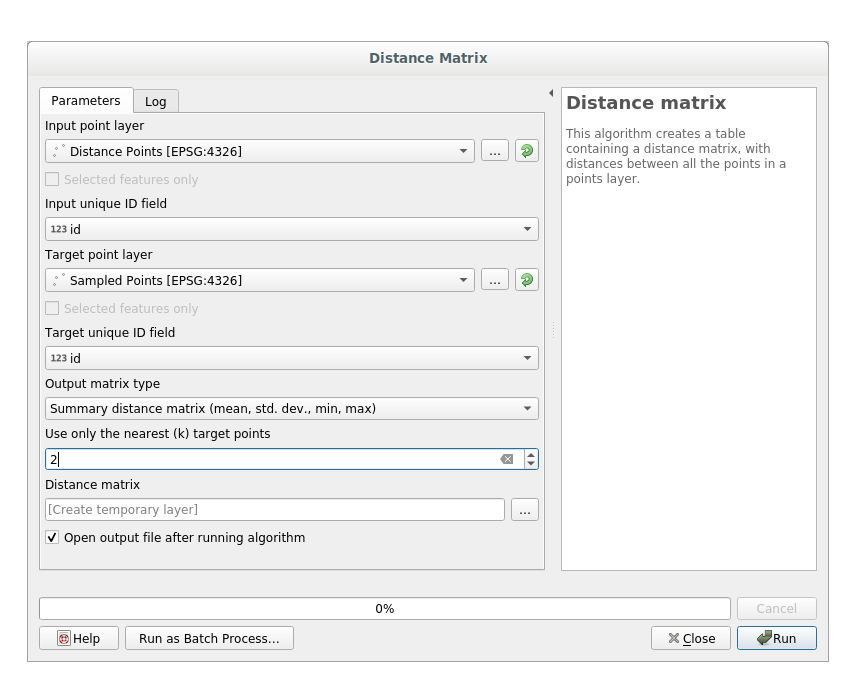4. If you want you can save the output layer as a file or just run the algorithm and save the temporary output layer in a second moment.

5. Click Run to generate the distance matrix layer.

6. Open the attribute table of the generated layer: values refer to the distances between the distance_points features and their two nearest points in the Sampled Points layer: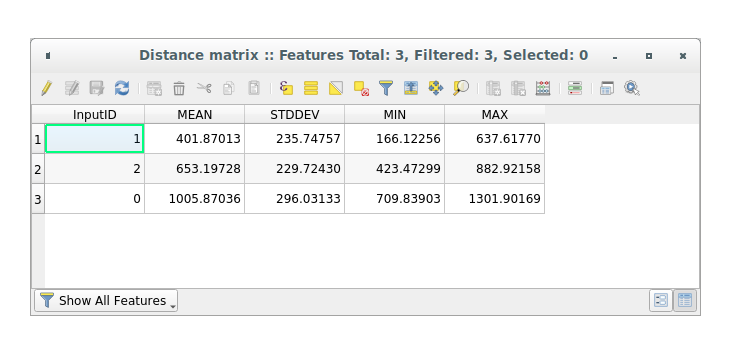With these parameters, the `Distance Matrix` tool calculates distance statistics for each point of the input layer with respect to the nearest points of the target layer. The fields of the output layer contains the mean, standard deviation, minimum and maximum for the distances to the nearest neighbors of the points in the input layer.

## 7.4.4.Follow Along: Nearest Neighbor Analysis (within layer)¶

To do a nearest neighbor analysis of a point layer:

1. Click on the menu item Vector analysis ‣ Nearest neighbor analysis.

2. In the dialog that appears, select the Random points layer and click Run.

3. The results will appear in the Processing Result Viewer Panel.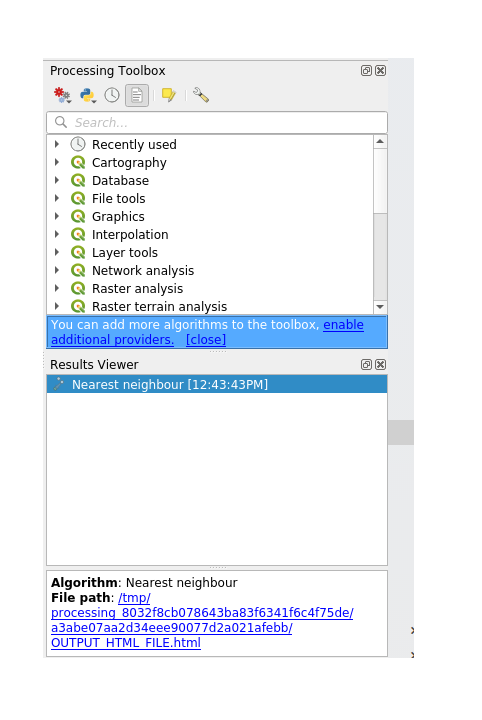4. Click on the blue link to open the `html` page with the results: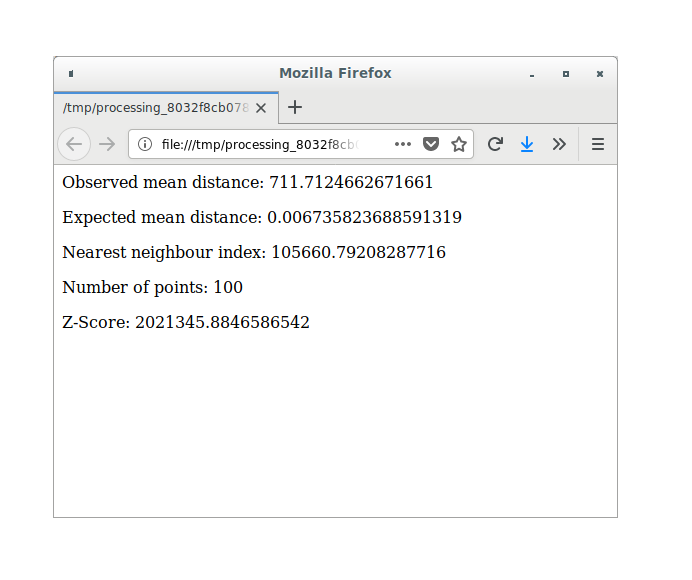## 7.4.5.Follow Along: Mean Coordinates¶

To get the mean coordinates of a dataset:

1. Click on the Vector analysis ‣ Mean coordinate(s) menu item.

2. In the dialog that appears, specify Random points as the input layer, but leave the optional choices unchanged.

3. Click Run.

Let’s compare this to the central coordinate of the polygon that was used to create the random sample.

1. Click on the Vector geometry ‣ Centroids menu item.

2. In the dialog that appears, select Bounding geometry as the input layer.

As you can see from the example below, the mean coordinates (pink point) and the center of the study area (in green) don’t necessarily coincide.

The centroid is the barycenter of the layer (the barycenter of a square is the center of the square) while the mean coordinates represent the average of all node coordinates.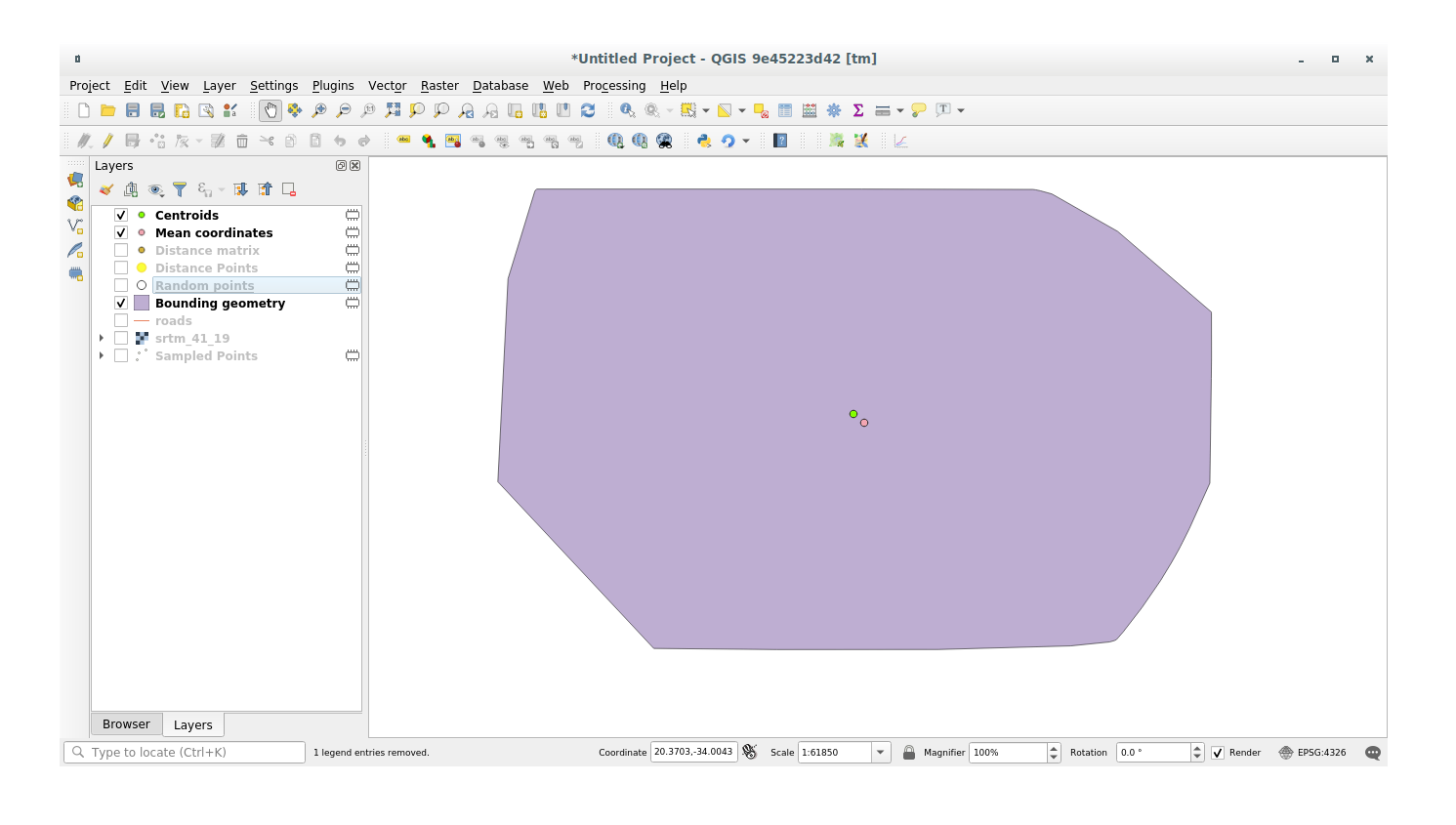## 7.4.6.Follow Along: Image Histograms¶

The histogram of a dataset shows the distribution of its values. The simplest way to demonstrate this in QGIS is via the image histogram, available in the Layer Properties dialog of any image layer (raster dataset).

1. In your Layers panel, right-click on the srtm_41_19 layer.

2. Select Properties.

3. Choose the tab Histogram. You may need to click on the Compute Histogram button to generate the graphic. You will see a graph describing the frequency of values in the image.

4. You can export it as an image: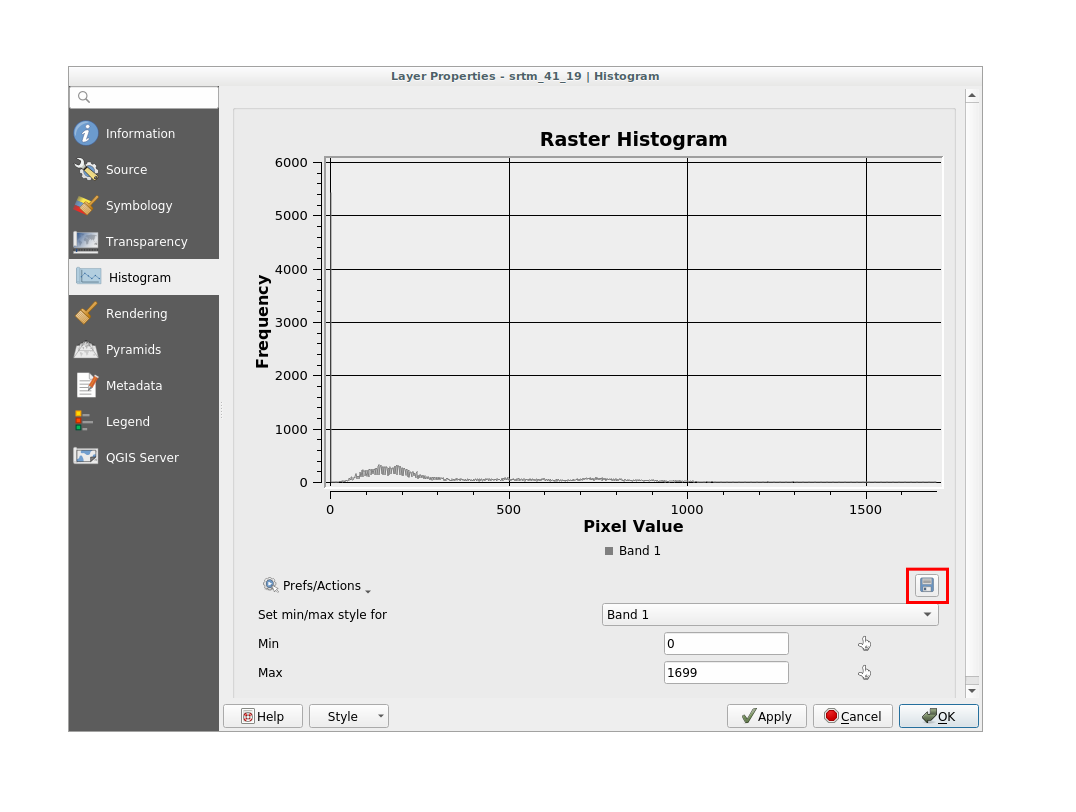5. Select the Information tab, you can see more detailed information of the layer.

The mean value is `332.8`, and the maximum value is `1699`! But those values don’t show up on the histogram. Why not? It’s because there are so few of them, compared to the abundance of pixels with values below the mean. That’s also why the histogram extends so far to the right, even though there is no visible red line marking the frequency of values higher than about `250`.

Note

If the mean and maximum values are not the same as those of the example, it can be due to the min/max value calculation. Open the Symbology tab and expand the Min / Max Value Settings menu. Choose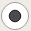Min / max and click on Apply.

Therefore, keep in mind that a histogram shows you the distribution of values, and not all values are necessarily visible on the graph.

## 7.4.7.Follow Along: Spatial Interpolation¶

Let’s say you have a collection of sample points from which you would like to extrapolate data. For example, you might have access to the Sampled points dataset we created earlier, and would like to have some idea of what the terrain looks like.

1. To start, launch the GDAL ‣ Raster analysis ‣ Grid (IDW with nearest neighbor searching) tool within Processing toolbox.

2. In the Point layer parameter, select Sampled points

3. Set `5.0` as the Weighting power

4. In the Advanced parameters set rvalue_1 for the Z value from field parameter

5. Finally click on Run and wait until the algorithm ends

6. Close the dialog

Here’s a comparison of the original dataset (left) to the one constructed from our sample points (right). Yours may look different due to the random nature of the location of the sample points.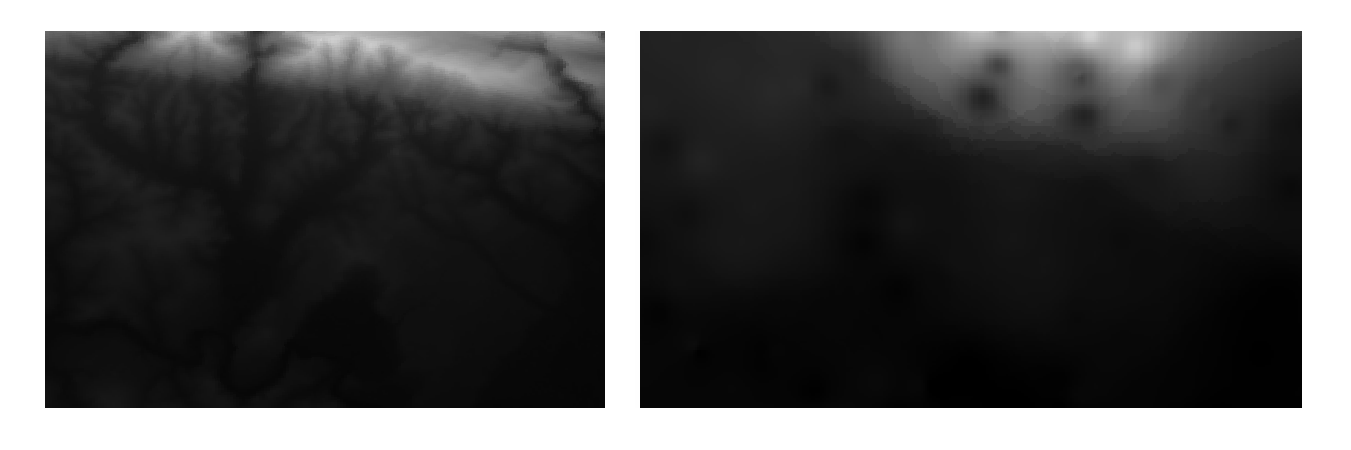As you can see, 100 sample points aren’t really enough to get a detailed impression of the terrain. It gives a very general idea, but it can be misleading as well.

## 7.4.8.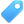Try Yourself Different interpolation methods¶

1. Use the processes shown above to create a new set of `10 000` random points.

Note

If the points amount is really big the processing time can take a long time.

2. Use these points to sample the original DEM.

3. Use the Grid (IDW with nearest neighbor searching) tool on this new dataset as above.

4. Set the Power and Smoothing to `5.0` and `2.0`, respectively.

The results (depending on the positioning of your random points) will look more or less like this: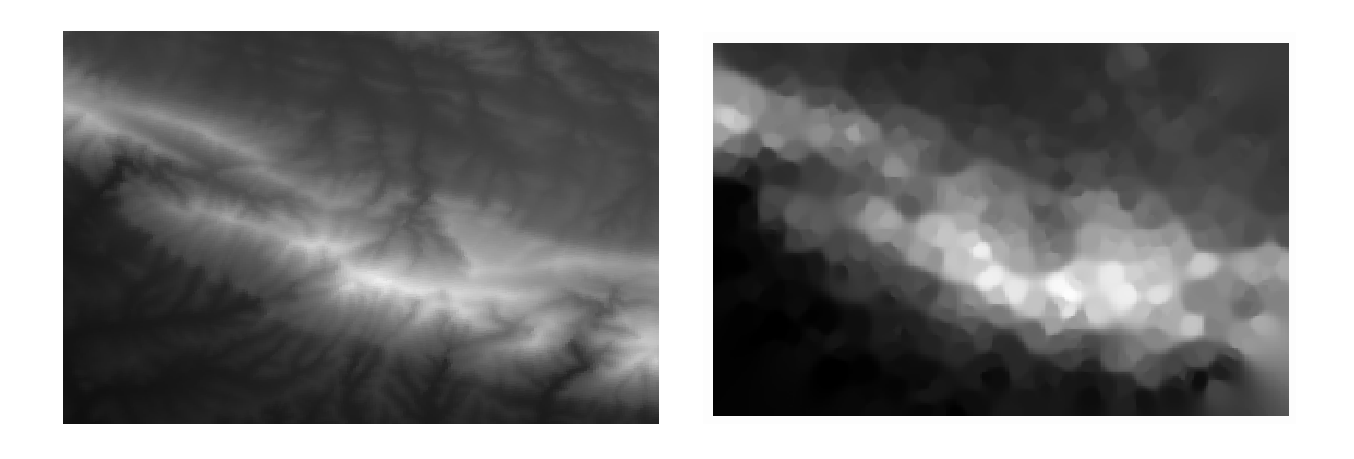This is a much better representation of the terrain, due to the much greater density of sample points. Remember, bigger samples give better results.

## 7.4.9. In Conclusion¶

QGIS allows many possibilities for analyzing the spatial statistical properties of datasets.

## 7.4.10. What’s Next?¶

Now that we’ve covered vector analysis, why not see what can be done with rasters? That’s what we’ll do in the next module!